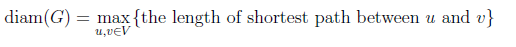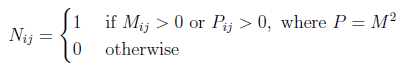Related Articles
GATE | GATE CS 2021 | Set 1 | Question 46
• Last Updated : 26 Feb, 2021

Let G=(V,E) be an undirected unweighted connected graph. The diameter of G is defined as:Let M be the adjacency matrix of G.

Define graph G2 on the same set of vertices with adjacency matrix N, whereWhich one of the following statements is true?
(A) diam(G2)≤⌈ diam(G)/2⌉
(B) ⌈ diam(G)/2⌉2)< diam(G)
(C) diam(G2) = diam(G)
(D) diam(G)< diam(G2)≤2 diam(G)